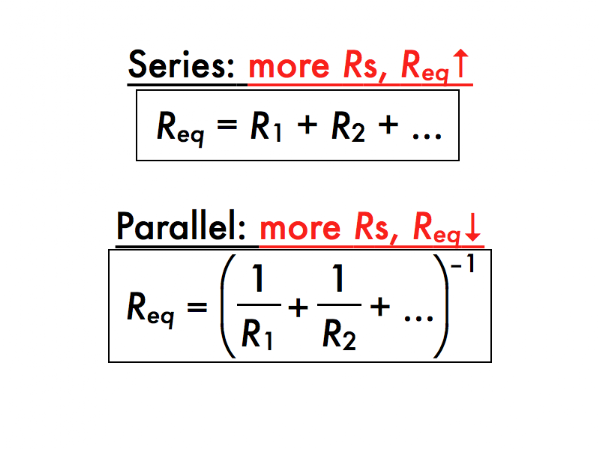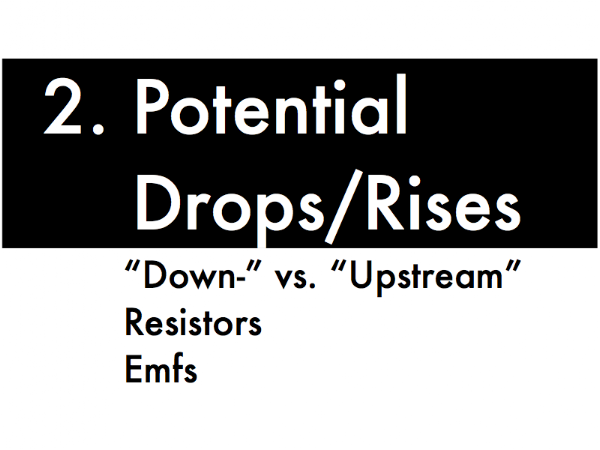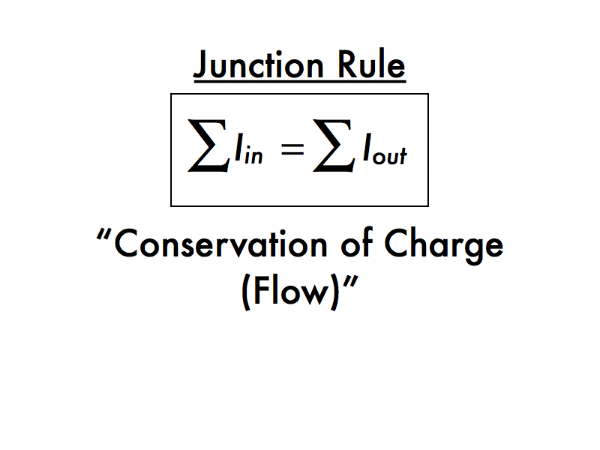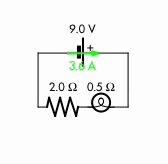## 20140321

### Presentation: circuit analysisPeople in a line, holding hands: check. On top of insulating materials: check. With a person at one end of the line touching an electric fence: check. Now with the person at the other side of the line, barefoot, in touch with the ground: priceless. (Video link: "Electric fence experiment ends as expected.")In this presentation we introduce the quantitative methods of analyzing circuits.First, calculating equivalent resistances for different configurations of resistors.The two basic configurations are where resistors are in series, literally "chained" together end-to-end such that current must pass through each resistor in sequence; or in parallel, where current can divide up such that portions of it can pass through several resistors simultaneously.

For most configurations of resistors, an equivalent resistance can be calculated, which represents the value of the single resistor that could replace them without affecting the rest of the circuit.The equivalent resistance of resistors in series is their arithmetic sum, such that adding another resistor in series would increase the equivalent resistance.For example, a 0.5 Ω light bulb and a 2.0 Ω resistor connected in series would have an equivalent resistance of 0.5 Ω + 2.0 Ω = 2.5 Ω.

In contrast, for resistors in parallel the equivalent resistance is calculated as the reciprocal of the sum of the reciprocals, such that adding another resistor in parallel would actually decrease the value of the equivalent resistance.So if a 0.5 Ω light bulb and 2.0 Ω resistor were instead connected in parallel with each other, the equivalent resistance would be:

Req = (1/(0.5 Ω) + 1/(2.0 Ω))-1 = (2.0 Ω-1 + 0.50 Ω-1)-1 = (2.5 Ω-1)-1 = 0.40 Ω.

We'll discuss a model of why equivalent resistances work so differently for series and parallel circuits later during lecture, especially after we learn how to apply Kirchhoff's rules in the last part of this presentation.Second, sign conventions for electric potential drops and rises in circuits.As with this kayaker flowing downstream, or this salmon jumping upstream, positive and negative signs will denote whether we are heading "down-" or "upstream" in an electrical circuit--losing or gaining electric potential (or voltage).Recall from Ohm's law (introduced in a previous presentation) that the electric potential (or voltage) difference ∆V between either end of a resistor can be related to the current I flowing through it, and the resistance R of the resistor.

Given that the current is flowing from left-to-right through this resistor, if we follow the current "downstream" from left-to-right, then the potential difference ∆V will drop by I·R, as the electric potential decreases in that direction. However, if we go from right-to-left, or "upstream" against the flow of current, then the potential difference ∆V will rise by I·R, as the electric potential increases in that direction.For an emf source (such as an ideal battery) with a potential difference of ε, we do not pay attention to the direction of current flowing through the battery, but instead watch how we move through the battery with respect to the positive (+) and negative (-) terminals, which respectively represent the higher and lower electrical potential ends of the battery.

If we go through the battery from the negative (-) to the positive (+) terminals, then the potential difference ∆V will be rise by ε, as the electric potential increases in that direction. However, if we go through the battery from the positive (+) to the negative (-) terminals, then the potential difference ∆V will drop by ε, as the electric potential decreases in that direction.

These sign conventions may seem obscure, but we will need to strictly obey them as we apply Kirchhoff's rules to electric circuits.Third, Kirchhoff's rules (finally).Kirchhoff's junction rule is a statement of current conservation (that is, conservation of charge flow per time). The sum of all currents flowing into a junction must equal the sum of all currents flowing out of that same junction.So basically, "what goes in must come out" (or if there are no junctions, "what comes through must go through"). This may seem like a simple concept, but it will be useful to enforce mathematically as we analyze electrical circuits, as in this tangled mess of wires.Let's illustrate the junction rule with a series circuit (with an ideal 9.0 V battery, 0.5 Ω light bulb, and 2.0 Ω resistor). Since there are no junctions for current to go elsewhere in this circuit, this means that the resulting current of 3.6 A must not only pass through the battery, but 3.6 A of current must also go through the wire segment on the right, through the light bulb and resistor, and through the wire segment on the left to get back to the battery. (Make sure you know how to derive the value of the current in this example from the equivalent resistance of this circuit and Ohm's law, as discussed in a previous presentation.)Now let's illustrate the junction rule with a parallel circuit (with an ideal 9.0 V battery, 0.5 Ω light bulb, and 2.0 Ω resistor). Note that there are two junctions. There is 22.5 A of current passing though the battery, and then through the wire segment on the right, and then this current splits up at the first junction, such that 18 A of current goes through the light bulb, and 4.5 A of current goes through the the battery. From the junction rule, the current going into this junction (22.5 A) must equal the currents leaving the junction (18 A + 4.5 A). The currents flowing through the light bulb (18 A) and the resistor (4.5 A) then merge at the second junction, and equal the current leaving the second junction (22.5 A), which is equal to the current going though the wire segment on the left to get back to the battery. (Again, make sure you know how to derive the current values in this example from the equivalent resistance of this circuit and Ohm's law.)Kirchhoff's loop rule is a statement of the conservation of electric potential (that is, electric potential energy per charge). If we follow a complete loop in an electrical circuit, such that we wind up back at our starting point, all of the electric potential rises added together must equal all of the electric potential drops added together.This is merely a consequence of having the same location as the initial and our final points as we travel around a complete loop in an electrical circuit--the voltage rises equals the voltage drops, for a "net zero" change in voltage when we arrive back at the starting point. Here Mrs. P-dog and her nephew and nieces run around a complete circuit (a playground set), running up the steps (gaining electric potential, as in traveling through an ideal battery from the (-) to the (+) terminals), and then sliding down the slide (losing electric potential, as in traveling "downstream" through a resistor), only to wind up again at their starting point, and subsequently making many, many round trips. For a complete loop, the gains equal the losses. Endless hours of entertainment. (Video link: " 20091008030.")Let's illustrate the loop rule with the series circuit from before. Topologically, there is only one loop in this circuit. Going clockwise around this loop, in the direction of current, the rise in voltage (going from the (-) to the (+) terminal of the battery) must be equal the drops in voltage (due to current flowing through the light bulb and the battery). Numerically the 9.0 V rise in voltage from the battery must equal the 1.8 V drop in voltage due to the light bulb together with the 7.2 V drop in voltage due to the resistor. When going around this complete loop of circuit (and for any loop), all of the voltage increases must be used up by the voltage decreases.

This means that because the light bulb and the battery are wired in series to the ideal battery, they will together "share" the 9.0 V from the battery. (However, all of the current from the battery passes through the light bulb and then passes through the battery.)

Now let's illustrate the loop rule with the parallel circuit from before. Topologically, there are three possible loops (can you see all three?) but we'll only consider the two "interesting" loops to travel counterclockwise around, in the direction of current.For the complete loop that includes the battery and the light bulb, going clockwise around this loop, in the direction of current, the 9.0 V rise in voltage (going from the (-) to the (+) terminal of the battery) must be equal to a 9.0 V drop in voltage (due to current flowing through the light bulb).Then for the complete loop that includes the battery and the resistor, going clockwise around this loop, in the direction of current, the 9.0 V rise in voltage (going from the (-) to the (+) terminal of the battery) must be equal to a 9.0 V drop in voltage (due to current flowing through the resistor).

This means that because the light bulb and the resistor are wired in parallel to the ideal battery, they will each use the full 9.0 V from the battery. (However, current from the battery splits up and is "shared" between the light bulb and the battery.)

For the people holding hands in contact with the electric fence at the start of this presentation, there is a voltage source that maintains the fence having a higher electric potential than the ground. With a complete circuit of people holding hands to complete the circuit from the electric fence to the ground, the current will then flow through them (losing electric potential as it flows "downstream"), and then through the voltage source and electric fence (gaining electric potential as it flows from the low potential (–) to the high potential (+) end), such that the voltage produced by the electric fence is exactly equal to the voltage lost as it current flows through the line of people. (Note that this is a simple current loop, as there are no junctions, such that according to Kirchhoff's junction rule the same amount of current is passing through each of these people!) In lecture we will look at examples of more complicated electrical circuits to analyze using Kirchhoff's rules.